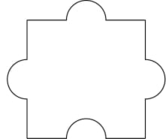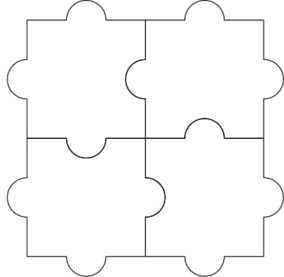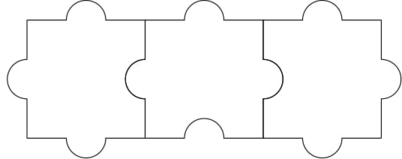Related Articles

# Check whether jigsaw puzzle solveable or not

• Difficulty Level : Easy
• Last Updated : 19 Apr, 2021

Given a special Jigsaw puzzle consisting of N rows and M columns all identical pieces. Every piece has three tabs and one blank. The task is to check if the puzzle is solvable by placing the pieces in such a way that the tab of one piece fits perfectly into a blank of other piece.Note: Rotate and Translate the pieces to solve the puzzle.

Examples:

Input: N = 2, M = 2
Output: YesInput: N = 1, M = 3
Output: YesApproach: The key observation in the problem is that:

• If the Puzzle has only one row or only one column. Then it is possible to solve the puzzle by placing a blank tab on that shared side itself.
• If the Puzzle has two rows and two columns. Then The puzzle is solvable by placing the blank Tabs in a circular chain.
• Otherwise, It is not possible to solve the Puzzle.

Below is the implementation of the above approach:

## C++

 `// C++ program for the above approach``#include ``using` `namespace` `std;` `// Function to check if the jigsaw``// Puzzle is solveable or not``void` `checkSolveable(``int` `n, ``int` `m)``{``  ` `    ``// Base Case``    ``if` `(n == 1 or m == 1)``        ``cout << ``"YES"``;``  ` `    ``// By placing the blank tabs``    ``// as a chain``    ``else` `if` `(m == 2 and n == 2)``        ``cout << ``"YES"``;``    ``else``        ``cout << ``"NO"``;``}`` ` `// Driver Code``int` `main()``{``    ``int` `n = 1, m = 3;``  ` `    ``checkSolveable(n, m);``}` `// This code is contributed by jana_sayantan`

## Java

 `// Java program for the above approach``import` `java.util.*;`` ` `class` `GFG{`` ` `// Function to check if the jigsaw``// Puzzle is solveable or not``static` `void` `checkSolveable(``int` `n, ``int` `m)``{``   ` `    ``// Base Case``    ``if` `(n == ``1` `|| m == ``1``)``       ``System.out.print(``"YES"``);``   ` `    ``// By placing the blank tabs``    ``// as a chain``    ``else` `if` `(m == ``2` `&& n == ``2``)``        ``System.out.print(``"YES"``);``    ``else``        ``System.out.print(``"NO"``);``}` `// Driver Code``public` `static` `void` `main(String[] args)``{``     ``int` `n = ``1``, m = ``3``;``   ` `    ``checkSolveable(n, m);``}``}` `// This code is contributed by sanjoy_62`

## Python

 `# Python program for the above approach` `# Function to check if the jigsaw``# Puzzle is solveable or not``def` `checkSolveable(n, m):` `    ``# Base Case``    ``if` `n ``=``=` `1` `or` `m ``=``=` `1``:``        ``print``(``"YES"``)``        ` `    ``# By placing the blank tabs``    ``# as a chain``    ``elif` `m ``=``=` `2` `and` `n ``=``=` `2``:``        ``print``(``"YES"``)``    ``else``:``        ``print``(``"NO"``)` `# Driver Code``if` `__name__ ``=``=` `"__main__"``:``    ``n ``=` `1``    ``m ``=` `3``    ``checkSolveable(n, m)`

## C#

 `// C# program for the above approach``using` `System;``  ` `class` `GFG{``  ` `// Function to check if the jigsaw``// Puzzle is solveable or not``static` `void` `checkSolveable(``int` `n, ``int` `m)``{``    ` `    ``// Base Case``    ``if` `(n == 1 || m == 1)``       ``Console.WriteLine(``"YES"``);``    ` `    ``// By placing the blank tabs``    ``// as a chain``    ``else` `if` `(m == 2 && n == 2)``        ``Console.WriteLine(``"YES"``);``    ``else``        ``Console.WriteLine(``"NO"``);``}`` ` `// Driver Code``public` `static` `void` `Main()``{``    ``int` `n = 1, m = 3;``  ` `    ``checkSolveable(n, m);``}``}` `// This code is contributed by susmitakundugoaldanga`

## Javascript

 ``
Output:
`YES`

Time Complexity: O(1)
Auxiliary Space: O(1)

Attention reader! Don’t stop learning now. Get hold of all the important DSA concepts with the DSA Self Paced Course at a student-friendly price and become industry ready.  To complete your preparation from learning a language to DS Algo and many more,  please refer Complete Interview Preparation Course.

In case you wish to attend live classes with experts, please refer DSA Live Classes for Working Professionals and Competitive Programming Live for Students.

My Personal Notes arrow_drop_up